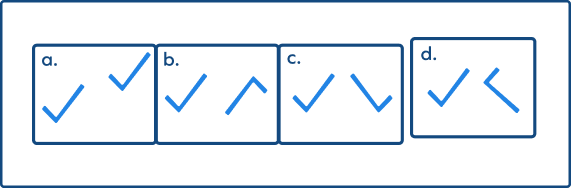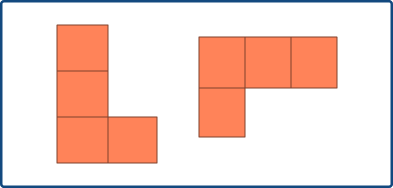# Slide in Maths

## Slide: Introduction

Slide is a transformation in which every point of the given shape or figure moves or slides in the same direction and also by the same distance. This transformation is also known as “translation.” So, what is a slide in math? To understand that, we must first learn about transformations.

Transformations are changes that are made to any shape.  Transformation in math is the method of transforming or changing the shape or size of an object using certain rules and methods. Flip (reflection), turn (rotation), and slide (translation) are the transformations or the positional changes we can make to a shape.

Note: After any of these transformations (turn, flip, or slide), the size, area, angles, and line lengths of the shape remain the same.

## What Is a Slide in Math?

Let us understand the definition of slide.

Slide or translation is a transformation in which every point of a plane figure simply moves in the same direction by the same distance in that very plane, without any rotation or resizing.

So, to undergo translation, every point of the shape must move

• same distance
• in the same direction

Every point of the given polygon has moved in the same direction and by the same distance. So, we can call it “slide” or “translation.”

## Examples of Slide

Let’s take a look at a few examples where a shape undergoes translation or slide transformation.

Example 1: The given shape has just moved to the right.

Example 2: The pencil has simply moved to the right. There’s no rotation or change in size.

Example 3: Sliding of a Square

## Slide on a Graph

Slides in geometry can be well-represented on a graph.

We can also represent the translation using an equation without a graph. For example, if the shape gets moved 5 units right, and 4 units up, we can write:

$(x, y) \rightarrow (x + 5, y + 4)$

It means that the x and y coordinates become “$x + 5$” and “$y + 4$” post translation.

Let’s see some examples.

Example 1: The following figure shows a slide or translation of a triangle. The shape has moved 2 units down and 2 units to the right.

$(x, y) \rightarrow (x + 2, y – 2)$

Example 2: Every point on the rectangle has moved 3 units to the right and 2 units down.

$(x, y) \rightarrow (x + 3, y – 2)$

## Conclusion

In this article, we learned about slide or translation transformation and discussed many examples using a graph. Let’s solve some examples to check our understanding.

## Solved Examples

1. Does the given image represent translation? Explain why or why not.

Solution:

Every point of the shape simply moved in the same direction by the same distance. So, it represents a slide.

2. Which figure represents the slide?

Solution:

Figure 1 represents a slide since the shape has simply moved to the right. In the case of figure 2, they are the mirror reflections of one another.

3. Identify the slide transformation.

Solution:

Figure 1 is the slide transformation of a triangle. Figure 2 shows rotation.

4. Does the given image represent a slide? Explain why or why not.

Solution: The given figure represents a slide because every point of the figure is moving in the same direction by the same distance in that very plane.

5. What is a slide? Draw a slide pair diagram.

Solution: A slide is a transformation in which every point of a plane figure moves in the same direction by the same distance in that very plane.

## Practice Problems

1

### Identify the figure representing slide or translation.a
b
c
d
CorrectIncorrect
In option a, the shape has simply moved, without any change in size or without any rotation.
2

### Which option shows an example of a slide?

$6 \rightarrow 9$
$9 \rightarrow 6$
$\text{x} \rightarrow \text{X}$
$\text{A} \rightarrow \text{A}$
CorrectIncorrect
Correct answer is: $\text{A} \rightarrow \text{A}$
Eliminate options where there is rotation or resizing involved, and identify the option where the shape simply moved from one point to another.
3

### The slide transformation is also known as

Flip
Turn
Translation
Rotation
CorrectIncorrect
Slide is also known as “translation.”
4

### The following image does not represent “slide” becauseThere is a change in size.
There is a change in shape.
The shape is rotated.
None of the above
CorrectIncorrect
Correct answer is: The shape is rotated.
This is not a translation because the shape is rotated.
5

### By translation, a shape moves 2 units to the left and 2 units down. Which option represents this transformation?

$(x, y) \rightarrow (x + 3, y - 2)$
$(x, y) \rightarrow (x - 2, y - 2)$
$(x, y) \rightarrow (x + 2, y - 2)$
$(x, y) \rightarrow (x - 2, y + 2)$
CorrectIncorrect
Correct answer is: $(x, y) \rightarrow (x - 2, y - 2)$
The shape moves 2 units to the left and 2 units down.
So, we can write $(x, y) \rightarrow (x - 2, y - 2)$